# Reviewing and Comparing of DSC Kinetics Methods

Differential scanning calorimetry (DSC) is used to measure heat flow into or out of a sample as it is exposed to a controlled thermal profile. DSC offers both quantitative and qualitative data about material transitions like crystallization, glass transition, melting, curing and decomposition.

For some of these transitions, DSC can not only provide the temperature at which transition takes place and the amount of heat involved, but also significant data about the kinetics or rate of the reaction. The advent of convenient computer-based data analysis programs has made it possible to obtain this kind of kinetic information.

Three software packages are currently offered by TA Instruments for DSC kinetic studies:

• Borchardt and Daniels
• ASTM E-698 Thermal Stability
• Isothermal Kinetics

Using these kinetic packages, it is possible to rapidly and automatically calculate reaction order (n and/or m), activation energy (Ea), pre-exponential factor (Z), and rate constant (k). It is also possible to use the kinetic parameters obtained for generating predictive thermal curves that can be used to examine the transition in terms of percent conversion, temperature and time. As there is no single approach satisfactory for all transitions, it is important to careful choose the right approach to obtain meaningful kinetic parameters. This article describes the theoretical basis for each kinetic approach and typical applications.

## Borchardt and Daniels

The Borchardt and Daniels (B/D) kinetics approach is used for the determination of activation energy (Ea), pre-exponential factor (Z), heat of reaction (DH), reaction order (n), and rate constant (k) from a single DSC scan. Originally this approach was explained by Borchardt and Daniels for solutions and was fine-tuned later for solids.

According to the Borchardt and Daniels approach, the reaction follows nth order kinetics and follows the general rate equation: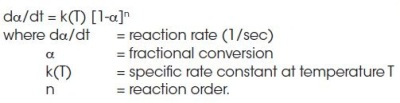(1)

The Borchardt and Daniels approach also takes on Arrhenius behavior: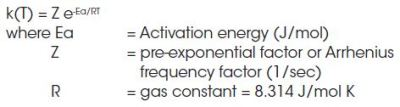(2)

By substituting equation (2) into equation (1), arranging again and then taking logarithm yields,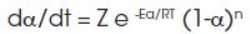(3)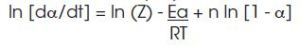(4)

It is possible to solve the equation with a multiple linear regression of the general form:

z = a + bx +cy where the two basic parameters (dα/dt and α) are determined from the DSC exotherm as observed in Figure 1 for an epoxy prepreg.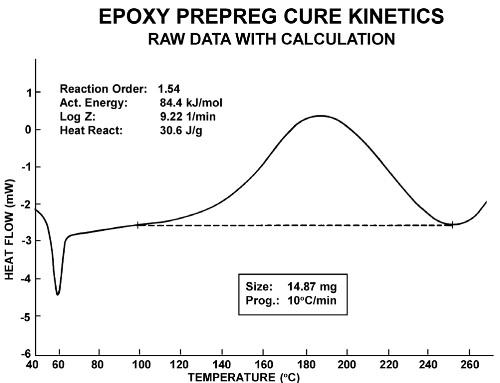Figure 1. Raw data with calculation

20 curve segments spaced uniformly by temperature are used for the determination. The first peak commences at 10% peak height and the last segment ends at 50% peak area.

If the second assumption is valid, a plot of ln[k(T)] versus 1/T (Arrhenius plot) from this data must be a straight line (see Figure 2). The pre-exponential factor(Z) and the activation energy (Ea) are obtained from the intercept and slope of this plot respectively.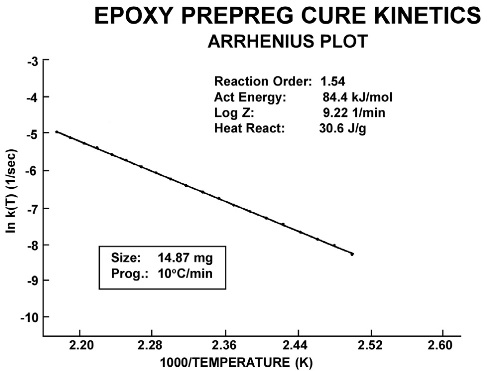Figure 2. Arrhenius plot

From the B/D kinetic modeling, two predictive curves are derived:

• Isothermal plots (Figure 3) – These provide the time and temperature conditions for a particular isothermal level.
• Isoconversion plots (Figure 4) – These offer time and temperature conditions for a particular conversion level.

Both the predictive plots are beneficial in the control and development of reaction conditions for achieving the preferred final product.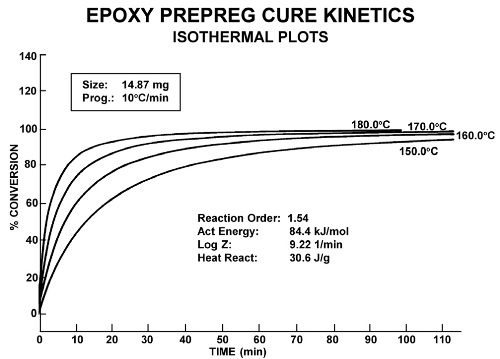Figure 3. Isothermal plots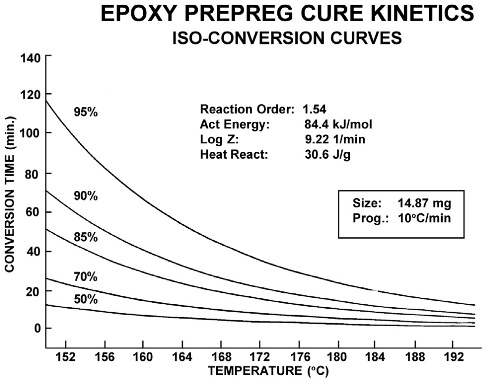Figure 4. Iso-conversion curves

There are two further experimental conditions for achieving desired results with the B/D approach.

• Mass loss must not occur during the reaction as sample weight is used for determining heat of reaction and is assumed constant in other determinations. In order to ensure a constant mass, the sample is arranged in a hermetically sealed plan and the weight is determined prior to and after the experiment.
• It is important that any thermal lag effects are avoided. This is accomplished by using heating rates which do not exceed 10°C/minute.

## ASTM E698 Thermal Stability

The variable program rate method of Ozawa is the basis of the ASTM E698 kinetics approach, requiring three or more experiments at different heating rates, normally between 1 and 10°C/minute.

Arrhenius behaviour and first order reaction kinetics are assumed in this approach, yielding the equation: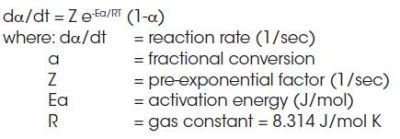(5)

since ß = dT/dt, where ß = heating rate

It is also possible to rearrange Equation 5 to yield: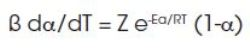(6)

The other assumptions of this technique are that the extent of reaction at the peak exotherm, αp, is constant and is not based on heating rate.

The data required for determining the activation energy (Ea), pre-exponential factor (Z), rate constant (k), and half-life (t1/2) is obtained from plotting the natural logarithm of the program rate versus the peak temperature (Figure 5).

Using a fourth degree polynomial, refinements of the approximated activation energy (Ea) are performed. Similar to the B/D approach, the determined kinetic parameters (Ea, Z, and k) can be used for generating isothermal and isoconversion predictive plots (Figures 3 and 4), and also half-life plots.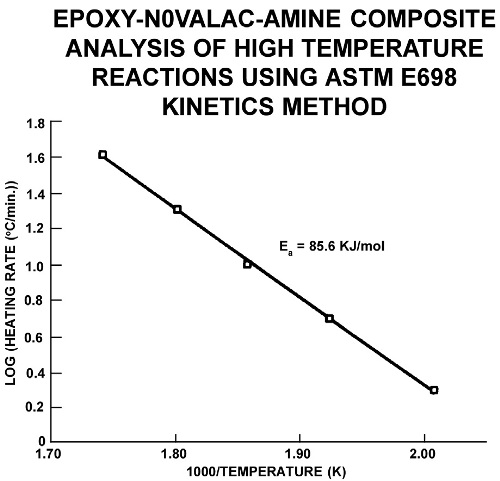Figure 5. Analysis of high-temperature reactions Isothermal

## Isothermal

The two dynamic kinetic approaches described previously are rapid and simple, however, they are not quantitatively applicable to autocatalyzed systems. Autocatalyzed systems are characterized by the formation of some intermediate species which markedly accelerates the reaction. For instance, epoxy systems used in industry are autocatalyzed systems.

It is possible to apply the isothermal kinetics approach to both autocatalyzed exothermic systems and nth order. It has to be noted that this approach is not used for modeling endothermic reactions or crystallization kinetics.

Equation 1 will be followed by an nth order reaction and an autocatalyzed reaction will follow the emperical relationship: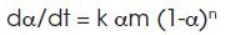(7)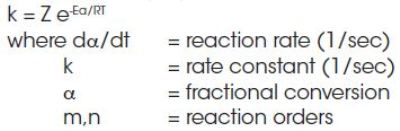(8)

Three or more isothermal experiments are required for this approach to generate kinetic parameters (Ea, Z, n, m, and k). As seen in Figure 6, the heat flow (dH/dt) and the partial heats (ΔHT ) are determined from the DSC isothermal exotherm using a reference theoretical heat of reaction, ΔHO. The determined values of α and dα/dt are then entered into the right kinetics model, either autocatalyzed or nth order and the best fit kinetic parameters are determined (Figure 7).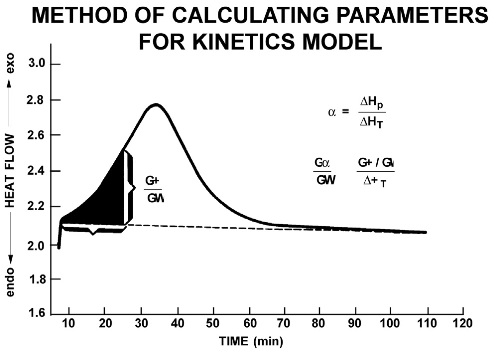Figure 6. Method for calculating parameters for kinetics models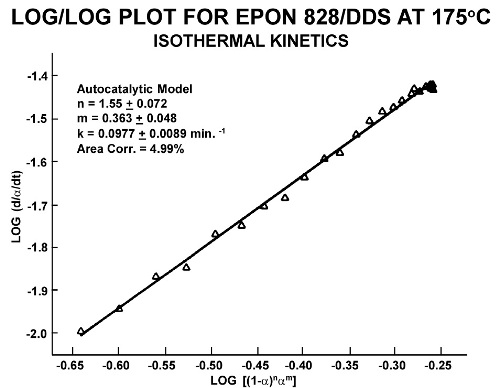Figure 7. Log/Log plot for EPON 828/DDS at 175°C

For deciding the optimum temperatures for the isothermal experiments, heat the material at 5°C/minute. Then select isothermal temperatures that fall between a temperature 10-20°C less than the onset of reaction and a temperature midway to the peak maximum (Figure 8).

When the actual isothermal experiments are performed, the cell is heated to the preferred isothermal temperature using an initial temperature program segment. The cell is opened quickly, the sample is loaded and the cell is again closed. Data collection commences when the displayed temperature is within 4°C of the desired isothermal temperature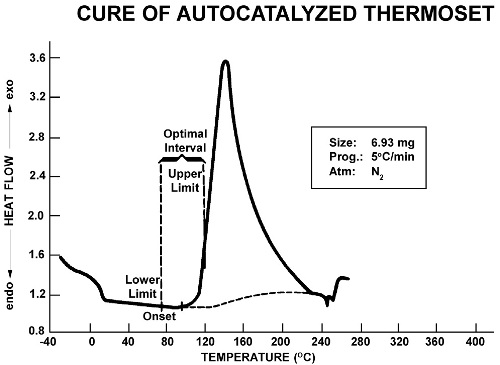Figure 8. Cure of autocatalyzed thermoset

## Method Comparison

Autocatalyzed Versus Nth Order Reactions

DSC helps determine if a particular exothermic material is autocatalyzed or follows nth order kinetics. A material sample is isothermally maintained at a very high temperature and the cure exotherm is monitored as a function of time. Materials following nth order kinetics will have the highest rate of heat evolution at time = 0, as shown in Figure 9, while the maximum heat evolution of an autocatalyzed material will be at 30 to 40 % of the reaction, as shown in Figure 6.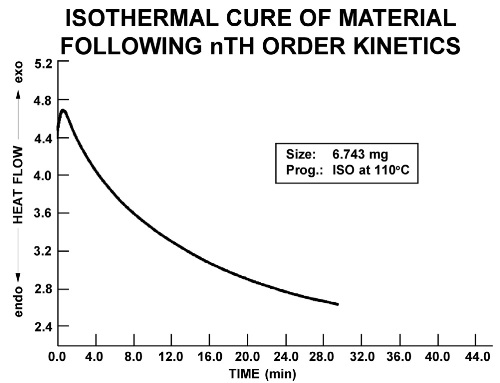Figure 9. Isothermal cure of material following nth order kinetics

Materials following nth order kinetics can be successfully modeled using the Borchardt and Daniels approach. However, It is important to note that the Borchardt and Daniels approach can still be used for non-quantitative assessment of autocatalyzed materials. In the event an adhesive is subjected to aging at room temperature, the impact of aging appears as a constant drop in the Borchardt and Daniels activation energy. Figure 10 shows this for a commercial autocatalyzed epoxy-amine adhesive aged at 65°C. A considerable change in the cure mechanism is believed to cause the sudden drop in activation energy after aging for 12h at 65°C. The glass transition of the aged thermoset also reflects this phenomenon.

The glass transition temperature (Tg) versus aging time at 65°C is plotted in Figure 11. It is observed that after 14h aging, there is a large increase in Tg. Hence for autocatalyzed thermosets, the glass transition temperature can be used as a quality control parameter.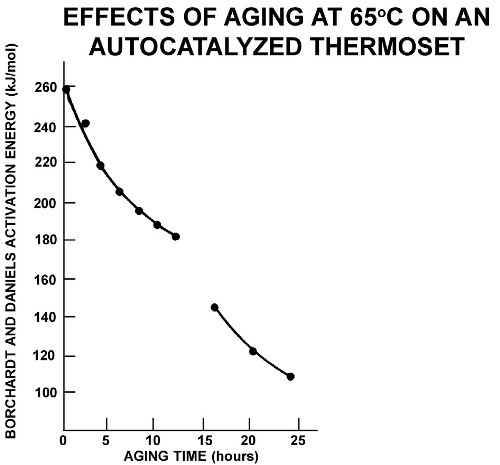Figure 10. Autocatalyzed thermoset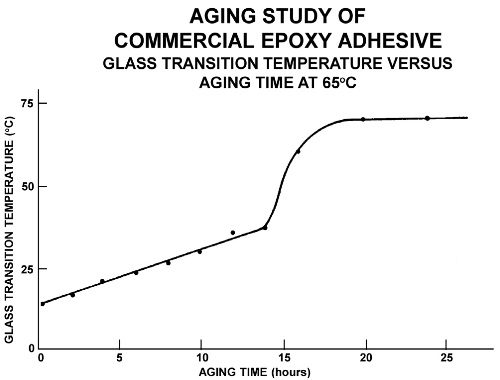Figure 11. Glass transition temperature vs. aging time

The ASTM E-698 method offers a precise assessment of the activation energy for autocatalyzed thermosets. As Z determination assumes nth order behavior, the pre-exponential factor (Z) may not be valid.

The 60-minute half-life test must be deployed for determining whether the ASTM E-698 approach successfully describes the thermoset kinetics.

In this method, the residual heat of cure of a sample that has been isothermally cured for 60 minutes at a temperature where half-life is about 2h is compared to the heat of cure (ΔHO) of a freshly prepared sample. It is important that the ratio of the exotherms (ΔH residual/ ΔHO) is 0.5 ± 0.05. If it is not, then the ASTM E-698 method does not successfully model the cure kinetics of the thermoset; probably due to an incorrect assessment of Z. Using an autocatalyzed cure-rate expression, a more precise estimation of the pre-exponential factor can be subsequently made.

## Dynamic Versus Isothermal Techniques

The Borchardt and Daniels approach is highly attractive approach as it necessitates only a single temperature programmed experiment. This is a significantly rapid technique and works perfectly for simple, first order reactions.

However, previous studies show that the B/D technique is not suited for the following cases:

• Overlapping reaction peaks
• Decomposition occurs during reaction
• Autocatalyzed reactions

ASTM or isothermal techniques can be used for more complex systems. The ASTM method is the only way to study reactions with irregular baselines, multiple exotherms or solvent effects. In these cases isothermal measurements and the B/D method cannot be relied upon.

Situations in which the ASTM method is not suitable include materials where isomerization changes take place at the reaction temperature or decomposition happens on melting. It is possible to identify these cases by a non-linear plot of log (program rate) versus peak temperature, or by a considerable difference between the data forecast and the isothermal aging confirmation.

Both the dynamic methods, ASTM and B/D take the assumption that the reaction being studied follows Arrhenius behavior and nth order kinetics. Reactions that don’t conform to these assumptions must be modeled using isothermal techniques.

Easy data interpretation and wider range of applicability are an additional benefit of isothermal methods. This method is suited for both nth order and autocatalytic reactions. For studying a new reaction where the reaction mechanism and the order are unknown, the isothermal method needs to be deployed. The isothermal experiments may consume time, however provide consistent kinetic parameters. This is due to the fact that the isothermal method introduces fewer experimental variables into a single measurement hence the scope for ambiguity in interpreting data is reduced.

## Conclusion

By modelling the kinetics of material reactions, the engineer and the scientist obtain valuable data for the following:

• Process development and prediction of optimum reaction temperatures
• Process control by optimization of reaction advancement or conversion

This kinetic data can be obtained using several DSC approaches. Figure 12 shows a chart for determining the right DSC method.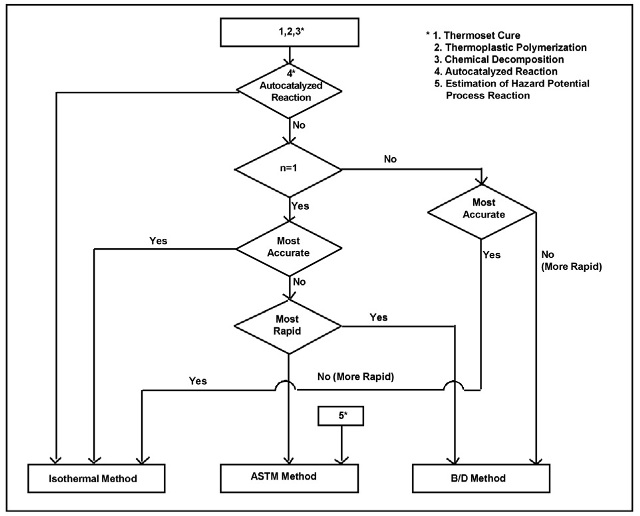Figure 12. Selecting a DSC methodThis information has been sourced, reviewed and adapted from materials provided by TA Instruments.

## Citations

• APA

TA Instruments. (2020, April 28). Reviewing and Comparing of DSC Kinetics Methods. AZoM. Retrieved on September 28, 2020 from https://www.azom.com/article.aspx?ArticleID=12105.

• MLA

TA Instruments. "Reviewing and Comparing of DSC Kinetics Methods". AZoM. 28 September 2020. <https://www.azom.com/article.aspx?ArticleID=12105>.

• Chicago

TA Instruments. "Reviewing and Comparing of DSC Kinetics Methods". AZoM. https://www.azom.com/article.aspx?ArticleID=12105. (accessed September 28, 2020).

• Harvard

TA Instruments. 2020. Reviewing and Comparing of DSC Kinetics Methods. AZoM, viewed 28 September 2020, https://www.azom.com/article.aspx?ArticleID=12105.